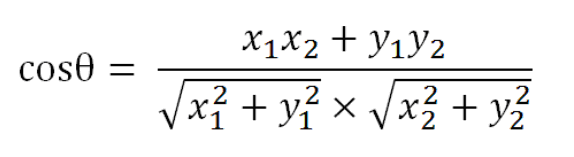• 为了找出相似的文章，需要用到"余弦相似性"（cosine similiarity）。下面，我举一个例子来说明，什么是"余弦相似性"。 为了简单起见，我们先从句子着手。 ? 1 2 句子A：我喜欢看电视，不喜欢看电影。 句子...
为了找出相似的文章，需要用到"余弦相似性"（cosine similiarity）。下面，我举一个例子来说明，什么是"余弦相似性"。
为了简单起见，我们先从句子着手。

?

1

2

句子A：我喜欢看电视，不喜欢看电影。

句子B：我不喜欢看电视，也不喜欢看电影。

请问怎样才能计算上面两句话的相似程度？
基本思路是：如果这两句话的用词越相似，它们的内容就应该越相似。因此，可以从词频入手，计算它们的相似程度。
第一步，分词。

?

1

2

句子A：我/喜欢/看/电视，不/喜欢/看/电影。

句子B：我/不/喜欢/看/电视，也/不/喜欢/看/电影。

第二步，列出所有的词。

?

1

我，喜欢，看，电视，电影，不，也。

第三步，计算词频。

?

1

2

句子A：我 1，喜欢 2，看 2，电视 1，电影 1，不 1，也 0。

句子B：我 1，喜欢 2，看 2，电视 1，电影 1，不 2，也 1。

第四步，写出词频向量。

?

1

2

句子A：[1, 2, 2, 1, 1, 1, 0]

句子B：[1, 2, 2, 1, 1, 2, 1]

到这里，问题就变成了如何计算这两个向量的相似程度。
我们可以把它们想象成空间中的两条线段，都是从原点（[0, 0, ...]）出发，指向不同的方向。两条线段之间形成一个夹角，如果夹角为0度，意味着方向相同、线段重合；如果夹角为90度，意味着形成直角，方向完全不相似；如果夹角为180度，意味着方向正好相反。因此，我们可以通过夹角的大小，来判断向量的相似程度。夹角越小，就代表越相似。以二维空间为例，上图的a和b是两个向量，我们要计算它们的夹角θ。余弦定理告诉我们，可以用下面的公式求得：假定a向量是[x1, y1]，b向量是[x2, y2]，那么可以将余弦定理改写成下面的形式：数学家已经证明，余弦的这种计算方法对n维向量也成立。假定A和B是两个n维向量，A是 [A1, A2, ..., An] ，B是 [B1, B2, ..., Bn] ，则A与B的夹角θ的余弦等于：使用这个公式，我们就可以得到，句子A与句子B的夹角的余弦。余弦值越接近1，就表明夹角越接近0度，也就是两个向量越相似，这就叫"余弦相似性"。所以，上面的句子A和句子B是很相似的，事实上它们的夹角大约为20.3度。
由此，我们就得到了"找出相似文章"的一种算法：

?

1

2

3

4

（1）使用TF-IDF算法，找出两篇文章的关键词；

（2）每篇文章各取出若干个关键词（比如20个），合并成一个集合，计算每篇文章对于这个集合中的词的词频（为了避免文章长度的差异，可以使用相对词频）；

（3）生成两篇文章各自的词频向量；

（4）计算两个向量的余弦相似度，值越大就表示越相似。

"余弦相似度"是一种非常有用的算法，只要是计算两个向量的相似程度，都可以采用它。
展开全文• 主要介绍了python代码如何实现余弦相似性计算,文中通过示例代码介绍的非常详细，对大家的学习或者工作具有一定的参考学习价值,需要的朋友可以参考下
• 主要介绍了余弦相似性计算及python代码实现过程解析,文中通过示例代码介绍的非常详细，对大家的学习或者工作具有一定的参考学习价值,需要的朋友可以参考下
• 根据余弦相似性原理判断两篇文章的相似程度。原理很简单，大致就是利用几个关键字组成一个特征向量，然后统计两篇文章各自对应的特征向量的值。最后计算两个特征矢量点积后的结果，即利用余弦值。如果值为1，表示两...
根据余弦相似性原理判断两篇文章的相似程度。原理很简单，大致就是利用几个关键字组成一个特征向量，然后统计两篇文章各自对应的特征向量的值。最后计算两个特征矢量点积后的结果，即利用余弦值。如果值为1，表示两篇文章高度相似。 这里使用了两篇关于神笔马良的短故事进行操作，对比两篇文章的相似性。 关键字是自己预先给定的，这里使用了：马良；弓；箭；画画；笔；财主；作为关键字。 主要公式：主程序代码如下：
%% 余弦相似性判断两篇文章的相似程度
clc;
clear all;
%% 录入关键字:马良；弓；箭；画画；笔；财主；
key_words = keyword_f();
%% 加载当前文件
%% 将关键字作为搜索词，统计当前文章中关键词出现的次数，得到特征矢量
% [关键词1，关键词2，关键词3]
M = size(key_words,1);
des_vector = zeros(1,M);
for i = 1:M
num_t = strfind(str1_now,key_words{1,1});
knum1 =  size(num_t,2)/size(str1_now,2);
des_vector(1,i) = knum1;
end

%% 加载待判断相似性的文件，可以是多个，这里仅用一个做例子
%% 将关键字作为搜索词，统计待搜索文章中关键词出现的次数，得到特征矢量
% [关键词1，关键词2，关键词3]
search_vector = zeros(1,M);
for i = 1:M
num_t = strfind(str_check,key_words{1,1});
knum1 =  size(num_t,2)/size(str_check,2);
search_vector(1,i) = knum1;
end

%% 进行余弦相似性检测
Mod1 = des_vector.*des_vector;
Mod2 = search_vector.*search_vector;
tempx = des_vector.*search_vector;
cosx = sum(tempx(:))/(sqrt(sum(Mod1(:)))*sqrt(sum(Mod2(:))))

function txt_str = load_file(file_name)
% 作用：读入文本文件，并删减掉无用的符号
% file_name:文件路径及名称
%   txt_str:删减后的文本字符串
%% 按照字符读取，中英字符，标点符号都算
% 空格，回车都不是字符，所以直接跳过，正好我们不需要这些。
fid = fopen(file_name);
A = textscan(fid,'%c');
% 转换成字符数组
B = A{1,1};
fclose(fid);

%% 将字符数组B中的标点符号和数字都删除掉。
M = size(B);
temp = [];
for i = 1:M
% 将所有是数字的字符索引记录下来
if B(i,1) == '0' || B(i,1) == '1' || B(i,1) == '2' || B(i,1) == '3' || B(i,1) == '4' || B(i,1) == '5' || B(i,1) == '6'...
|| B(i,1) == '7' || B(i,1) == '8' || B(i,1) == '9'
temp = [temp;i];
end
% 将常用的标点符号索引记录下来
if (B(i,1)=='，') ||  (B(i,1)==',') || (B(i,1)=='。') || (B(i,1)=='、') || (B(i,1)=='；') || (B(i,1)=='：')|| (B(i,1)==':') ...
|| (B(i,1)=='？') || (B(i,1)=='“') || (B(i,1)=='”') || (B(i,1)=='【') || (B(i,1)=='】')
temp = [temp;i];
end
% 将常用的特殊符号索引记录下来
if (B(i,1)=='+') || (B(i,1)=='—') ||  (B(i,1)=='-') || (B(i,1)=='=') || (B(i,1)=='*') ...
||  (B(i,1)=='&') || (B(i,1)=='%') ||  (B(i,1)=='￥') ||  (B(i,1)=='(')||  (B(i,1)==')')...
|| (B(i,1)=='#') ||  (B(i,1)=='@') || (B(i,1)=='!') ||  (B(i,1)=='{')||  (B(i,1)=='}')...
||  (B(i,1)=='\')||  (B(i,1)=='/')||  (B(i,1)=='[')||  (B(i,1)==']')||  (B(i,1)=='（')...
||  (B(i,1)=='）')
temp = [temp;i];
end
% 将26 个小写字母索引录下来
if (B(i,1)=='a') || (B(i,1)=='b') ||  (B(i,1)=='c') || (B(i,1)=='d') || (B(i,1)=='e') ...
||  (B(i,1)=='f') || (B(i,1)=='g') ||  (B(i,1)=='h') ||  (B(i,1)=='i')||  (B(i,1)=='j')...
|| (B(i,1)=='k') ||  (B(i,1)=='l') || (B(i,1)=='m') ||  (B(i,1)=='n')||  (B(i,1)=='o')...
||  (B(i,1)=='p')||  (B(i,1)=='q')||  (B(i,1)=='r')||  (B(i,1)=='s')||  (B(i,1)=='t')...
||  (B(i,1)=='u')||  (B(i,1)=='v')||  (B(i,1)=='w')||  (B(i,1)=='x')||  (B(i,1)=='y') || (B(i,1)=='z')
temp = [temp;i];
end

% 将26 个大写写字母索引录下来
if (B(i,1)=='A') || (B(i,1)=='B') ||  (B(i,1)=='C') || (B(i,1)=='D') || (B(i,1)=='E') ...
||  (B(i,1)=='F') || (B(i,1)=='G') ||  (B(i,1)=='H') ||  (B(i,1)=='I')||  (B(i,1)=='J')...
|| (B(i,1)=='K') ||  (B(i,1)=='L') || (B(i,1)=='M') ||  (B(i,1)=='N')||  (B(i,1)=='O')...
||  (B(i,1)=='P')||  (B(i,1)=='Q')||  (B(i,1)=='R')||  (B(i,1)=='S')||  (B(i,1)=='T')...
||  (B(i,1)=='U')||  (B(i,1)=='V')||  (B(i,1)=='W')||  (B(i,1)=='X')||  (B(i,1)=='Y') || (B(i,1)=='Z')
temp = [temp;i];
end

end

N = size(temp,1);
for j = temp
B(j,:) = [];    % 删除所有被标记的行
end

txt_str = B';
end

keyword_f.m函数代码：
function output = keyword_f()
% 作用：提示输入关键字，并将关键字拆分放置

% 录入字符
disp('关键字输入示例：');
disp('    你好；祖国；山水；房子；');
Key_Words = input('请输入所需要的关键字：','s');

%% 拆分出关键字
% 先找出分号的位置
ind(1,1) = 0;
M = size(Key_Words,2);
for i = 1:M
if Key_Words(1,i) == '；'
ind = [ind;i];
end
end
% 根据分号的位置进行关键词拆分，结果保存在KWSP
M = size(ind,1);
for i = 1:M-1
KWSP{i,1} = Key_Words(1,ind(i)+1:ind(i+1)-1);
end

output = KWSP;
end

输出结果：1，表示非常相似。
展开全文MATLAB
• C#源码,演示字符串相似度 编辑距离 余弦相似性 SimHash算法
• TF-IDF结合余弦相似性 判断文章相似性
 摘自：阮一峰的网络日志(http://www.ruanyifeng.com/blog/2013/03/cosine_similarity.html)
为了找出相似的文章，需要用到"余弦相似性"（cosine similiarity）。下面，我举一个例子来说明，什么是"余弦相似性"。
为了简单起见，我们先从句子着手。

句子A：我喜欢看电视，不喜欢看电影。
句子B：我不喜欢看电视，也不喜欢看电影。

请问怎样才能计算上面两句话的相似程度？
基本思路是：如果这两句话的用词越相似，它们的内容就应该越相似。因此，可以从词频入手，计算它们的相似程度。
第一步，分词。

句子A：我/喜欢/看/电视，不/喜欢/看/电影。
句子B：我/不/喜欢/看/电视，也/不/喜欢/看/电影。

第二步，列出所有的词。

我，喜欢，看，电视，电影，不，也。

第三步，计算词频。

句子A：我 1，喜欢 2，看 2，电视 1，电影 1，不 1，也 0。
句子B：我 1，喜欢 2，看 2，电视 1，电影 1，不 2，也 1。

第四步，写出词频向量。

句子A：[1, 2, 2, 1, 1, 1, 0]
句子B：[1, 2, 2, 1, 1, 2, 1]

到这里，问题就变成了如何计算这两个向量的相似程度。
我们可以把它们想象成空间中的两条线段，都是从原点（[0, 0, ...]）出发，指向不同的方向。两条线段之间形成一个夹角，如果夹角为0度，意味着方向相同、线段重合；如果夹角为90度，意味着形成直角，方向完全不相似；如果夹角为180度，意味着方向正好相反。因此，我们可以通过夹角的大小，来判断向量的相似程度。夹角越小，就代表越相似。以二维空间为例，上图的a和b是两个向量，我们要计算它们的夹角θ。余弦定理告诉我们，可以用下面的公式求得：假定a向量是[x1, y1]，b向量是[x2, y2]，那么可以将余弦定理改写成下面的形式：数学家已经证明，余弦的这种计算方法对n维向量也成立。假定A和B是两个n维向量，A是 [A1, A2, ..., An] ，B是 [B1, B2, ..., Bn] ，则A与B的夹角θ的余弦等于：使用这个公式，我们就可以得到，句子A与句子B的夹角的余弦。余弦值越接近1，就表明夹角越接近0度，也就是两个向量越相似，这就叫"余弦相似性"。所以，上面的句子A和句子B是很相似的，事实上它们的夹角大约为20.3度。
由此，我们就得到了"找出相似文章"的一种算法：

（1）使用TF-IDF算法，找出两篇文章的关键词；
（2）每篇文章各取出若干个关键词（比如20个），合并成一个集合，计算每篇文章对于这个集合中的词的词频（为了避免文章长度的差异，可以使用相对词频）；
（3）生成两篇文章各自的词频向量；
（4）计算两个向量的余弦相似度，值越大就表示越相似。

"余弦相似度"是一种非常有用的算法，只要是计算两个向量的相似程度，都可以采用它。
展开全文tf idf
• 主要为大家详细介绍了TF-IDF与余弦相似性的应用，找出相似文章，具有一定的参考价值，感兴趣的小伙伴们可以参考一下
• ## 余弦相似性计算

千次阅读 2016-03-09 15:35:44
为了找出相似的文章，需要用到"余弦相似性"（cosine similiarity）。下面，我举一个例子来说明，什么是"余弦相似性"。 为了简单起见，我们先从句子着手。 　句子A：我喜欢看电视，不喜欢看电影。 　句子B
今天，我们再来研究另一个相关的问题。有些时候，除了找到关键词，我们还希望找到与原文章相似的其他文章。比如，"Google新闻"在主新闻下方，还提供多条相似的新闻。为了找出相似的文章，需要用到"余弦相似性"（cosine similiarity）。下面，我举一个例子来说明，什么是"余弦相似性"。
为了简单起见，我们先从句子着手。

句子A：我喜欢看电视，不喜欢看电影。
句子B：我不喜欢看电视，也不喜欢看电影。

请问怎样才能计算上面两句话的相似程度？
基本思路是：如果这两句话的用词越相似，它们的内容就应该越相似。因此，可以从词频入手，计算它们的相似程度。
第一步，分词。

句子A：我/喜欢/看/电视，不/喜欢/看/电影。
句子B：我/不/喜欢/看/电视，也/不/喜欢/看/电影。

第二步，列出所有的词。

我，喜欢，看，电视，电影，不，也。

第三步，计算词频。

句子A：我 1，喜欢 2，看 2，电视 1，电影 1，不 1，也 0。
句子B：我 1，喜欢 2，看 2，电视 1，电影 1，不 2，也 1。

第四步，写出词频向量。

句子A：[1, 2, 2, 1, 1, 1, 0]
句子B：[1, 2, 2, 1, 1, 2, 1]

到这里，问题就变成了如何计算这两个向量的相似程度。
我们可以把它们想象成空间中的两条线段，都是从原点（[0, 0, ...]）出发，指向不同的方向。两条线段之间形成一个夹角，如果夹角为0度，意味着方向相同、线段重合；如果夹角为90度，意味着形成直角，方向完全不相似；如果夹角为180度，意味着方向正好相反。因此，我们可以通过夹角的大小，来判断向量的相似程度。夹角越小，就代表越相似。以二维空间为例，上图的a和b是两个向量，我们要计算它们的夹角θ。余弦定理告诉我们，可以用下面的公式求得：假定a向量是[x1, y1]，b向量是[x2, y2]，那么可以将余弦定理改写成下面的形式：数学家已经证明，余弦的这种计算方法对n维向量也成立。假定A和B是两个n维向量，A是 [A1, A2, ..., An] ，B是 [B1, B2, ..., Bn] ，则A与B的夹角θ的余弦等于：使用这个公式，我们就可以得到，句子A与句子B的夹角的余弦。余弦值越接近1，就表明夹角越接近0度，也就是两个向量越相似，这就叫"余弦相似性"。所以，上面的句子A和句子B是很相似的，事实上它们的夹角大约为20.3度。
由此，我们就得到了"找出相似文章"的一种算法：

（1）使用TF-IDF算法，找出两篇文章的关键词；
（2）每篇文章各取出若干个关键词（比如20个），合并成一个集合，计算每篇文章对于这个集合中的词的词频（为了避免文章长度的差异，可以使用相对词频）；
（3）生成两篇文章各自的词频向量；
（4）计算两个向量的余弦相似度，值越大就表示越相似。

"余弦相似度"是一种非常有用的算法，只要是计算两个向量的相似程度，都可以采用它。

原文地址： http://www.ruanyifeng.com/blog/2013/03/cosine_similarity.html 
展开全文• 请点击下面链接查看转载文章： TF-IDF与余弦相似性的应用
• 上一次，我用TF-IDF算法自动提取关键词。...为了找出相似的文章，需要用到"余弦相似性"（cosine similiarity）。下面，我举一个例子来说明，什么是"余弦相似性"。 为了简单起见，我们先从句子着手机器学习 数据挖掘
•TF-IDF
• 主要为大家详细介绍了TF-IDF与余弦相似性的应用，具有一定的参考价值，感兴趣的小伙伴们可以参考一下
•机器学习 spark
• 余弦相似性获取文章相似度的java实现,tf-idf算法实现文件相似度
• 关于余弦相似性算法，大家可以看这篇文章：TF-IDF与余弦相似性的应用（二） 这篇文章是基于分词的，里面有关于分词的知识。 其重点在于余弦算法： 这个算法的场景是这样的： 如何从一系列的字符串列表中找出一...java 算法
• 相似性检查器 使用余弦相似度获取相似度图或分数 例子 从查询图像点击猫的耳朵。 然后您可以从关键图像获取相似度图。 左图是真实的余弦相似度值，右图是热图图像。 如果使用与查询图像相同的关键图像，则可以...Python
• Faiss 相似度搜索使用余弦相似性 flyfish Faiss提供了faiss.METRIC_INNER_PRODUCT 和faiss.METRIC_L2 只需要我们代码加上normalize_L2 IndexIVFFlat在参数选择时，使用faiss.METRIC_INNER_PRODUCT 为了验证正确性，...
• 余弦相似性(余弦相似度)：通过计算两个向量的夹角余弦值来评估他们的相似性。 0度角的余弦值是1，而其他任何角度的余弦值都不大于1；并且其最小值是-1。从而两个向量之间的角度的余弦值确定两个向量是否大致指向...
• Moviebox：基于内容的机器学习推荐系统利用tf-idf和余弦相似性算法
• 为了找出相似的文章，需要用到"余弦相似性"（cosine similiarity）。下面，我举一个例子来说明，什么是"余弦相似性"。 为了简单起见，我们先从句子着手。 句子A：我喜欢看电视，不喜欢看电影。 句子B：我不喜欢看...TF-IDF 数据分析
• 深度学习基础 - 从余弦定理到余弦相似性 flyfish 首先有两个向量,a,b 把这两个向量放到二维平面直角坐标系 在平面直角坐标系中，向量的坐标由一对数字构成。这一对数字表示如何从向量的起点即坐标原点出发到达向量的...
• 余弦相似性简介 余弦相似性相关知识请参考：百度百科(余弦相似度) 二维余弦相似度计算公式：cos(θ)cos(\theta)cos(θ)=x1x2+y1y2x12+y12∗x22+y22{x_1x_2+y_1y_2}\over{\sqrt {x_1^2+y_1^2}*\sqrt {x_2^2+y_2^2}}x...python 数据分析 numpy 机器学习 人工智能
• 皮尔逊相关系数 ...余弦相似性 皮尔逊相关系数与余弦相似性的关系 皮尔逊相关系数就是减去平均值(中心化)后做余弦相似性 转载于:https://www.cnblogs.com/tracer-dhy/p/5808323.html...
• 为了找出相似的文章，需要用到"余弦相似性"（cosine similiarity）。下面，我举一个例子来说明，什么是"余弦相似性"。 为了简单起见，我们先从句子着手。 　句子A：我喜欢看电视，不喜欢看电影。 　句子B......